Finding General Solutions

Chapter 3 Class 11 Trigonometric Functions
Concept wise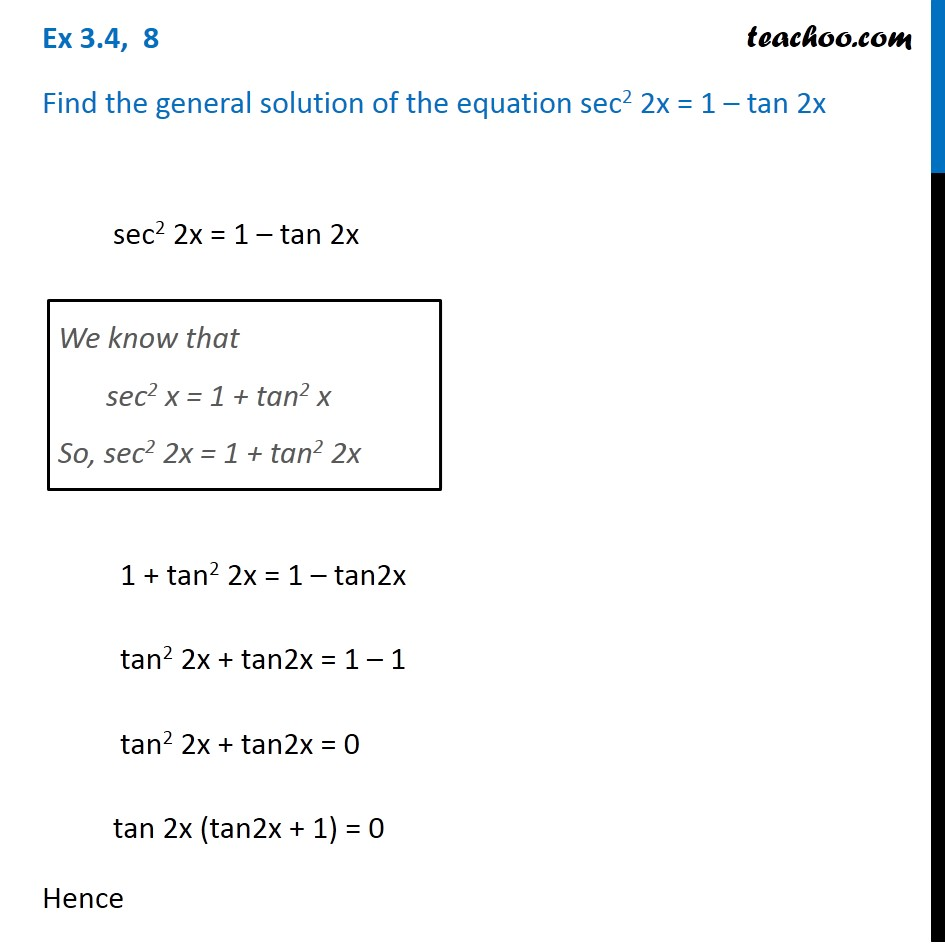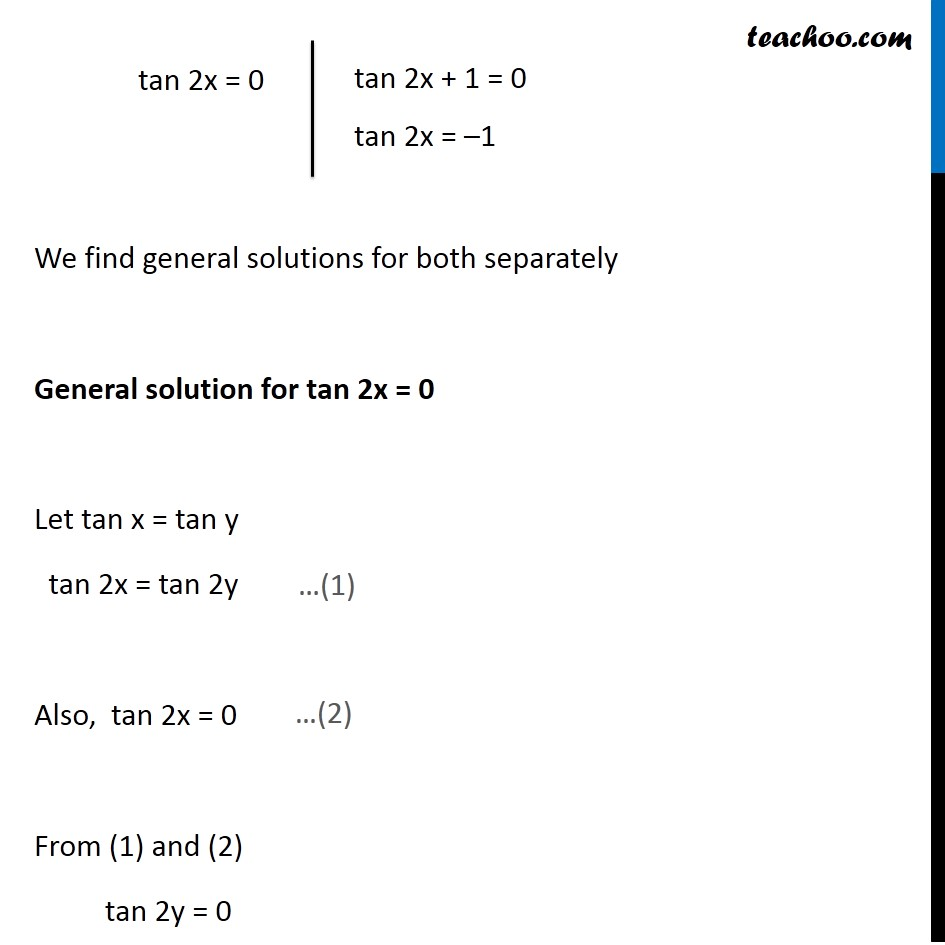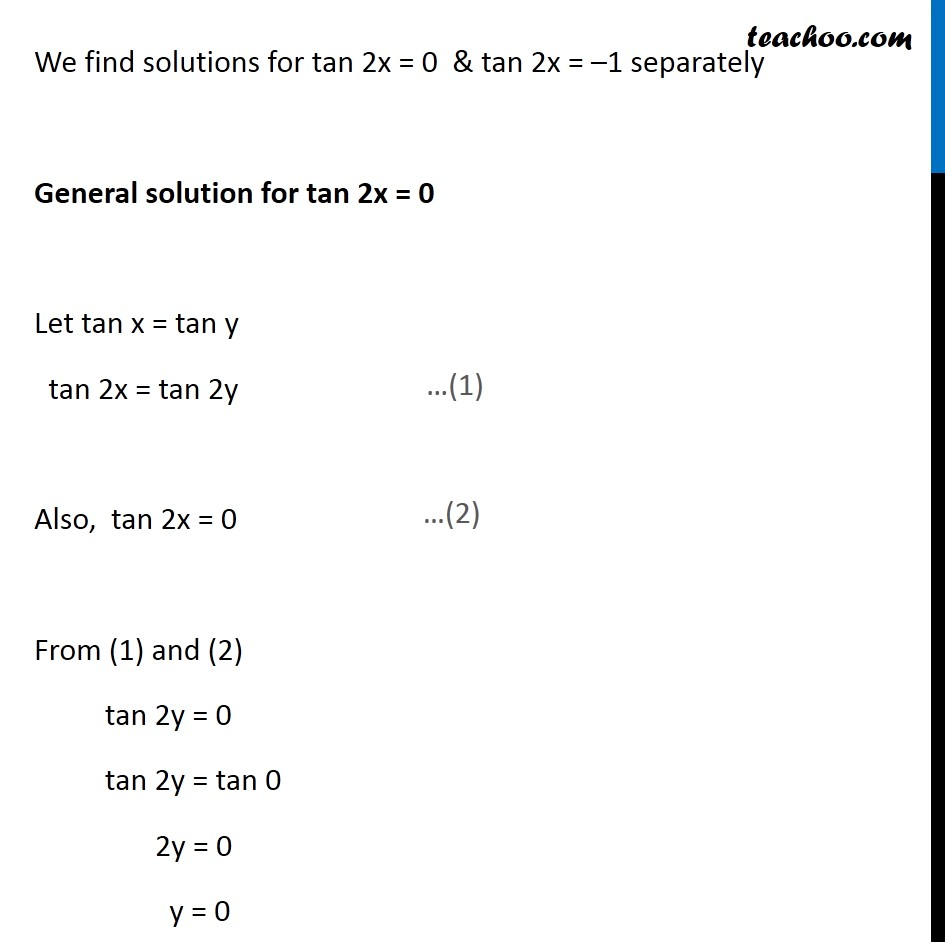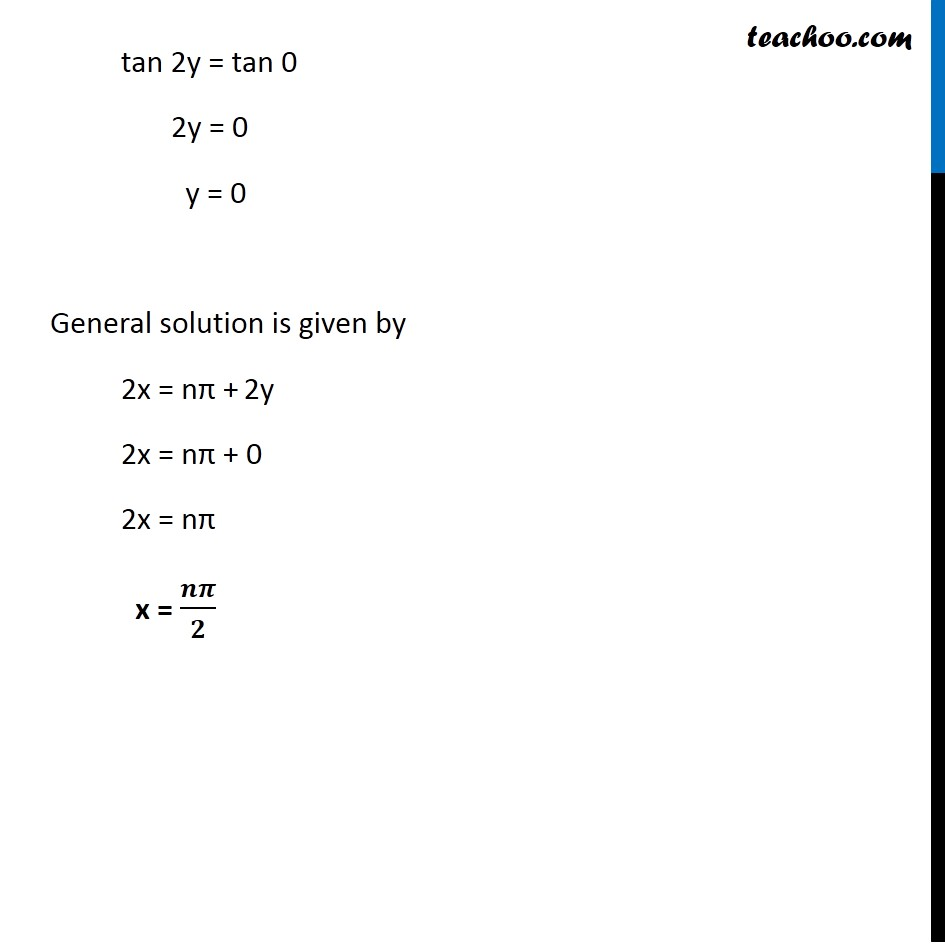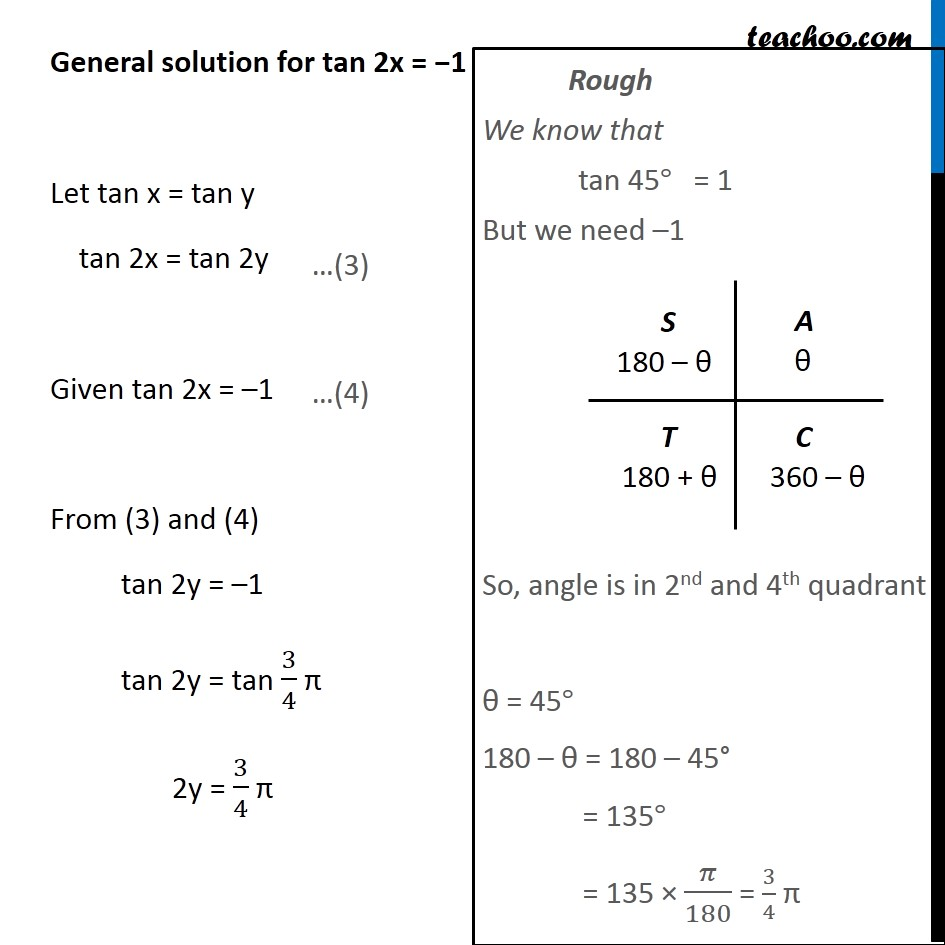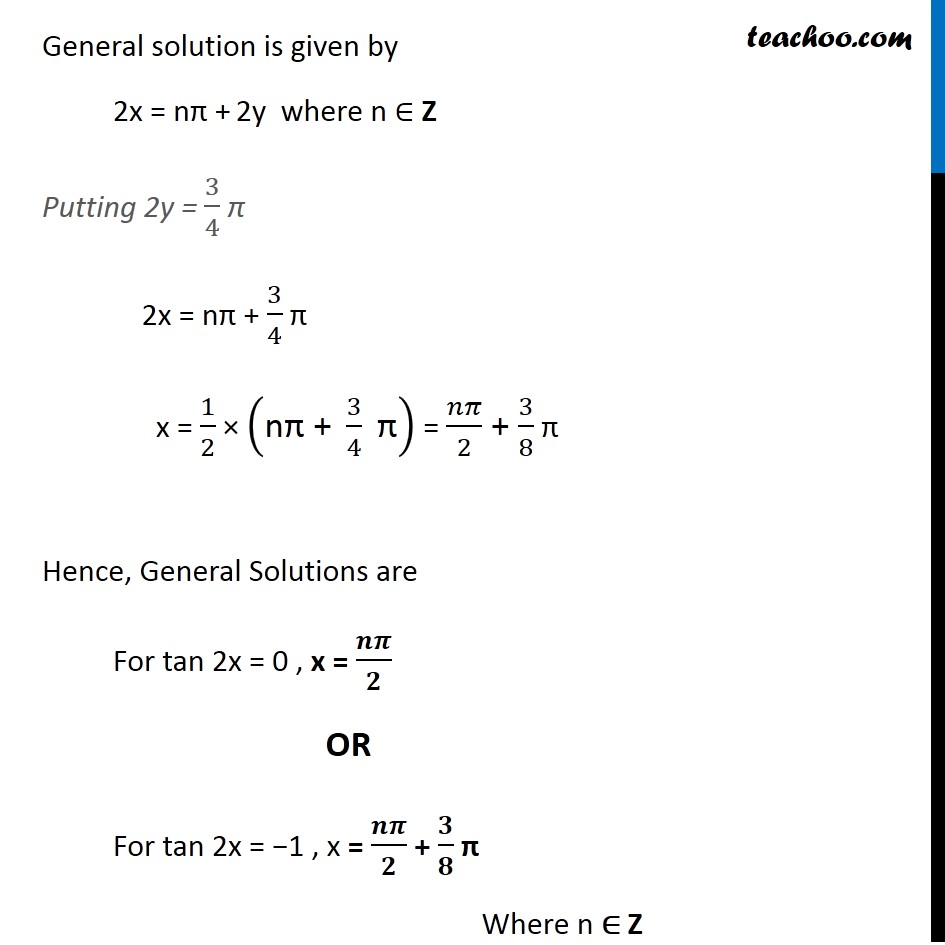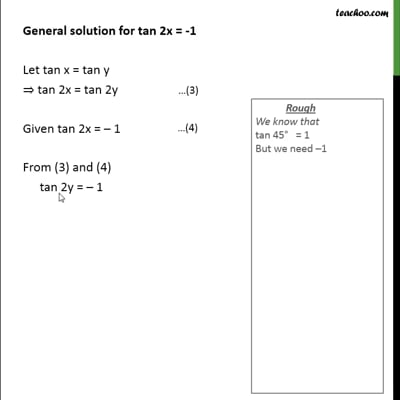This video is only available for Teachoo black users

Introducing your new favourite teacher - Teachoo Black, at only ₹83 per month

### Transcript

Ex 3.4, 8 Find the general solution of the equation sec2 2x = 1 – tan 2x sec2 2x = 1 – tan 2x 1 + tan2 2x = 1 – tan2x tan2 2x + tan2x = 1 – 1 tan2 2x + tan2x = 0 tan 2x (tan2x + 1) = 0 Hence We know that sec2 x = 1 + tan2 x So, sec2 2x = 1 + tan2 2x tan 2x = 0 tan 2x + 1 = 0 tan 2x = –1 We find general solutions for both separately General solution for tan 2x = 0 Let tan x = tan y tan 2x = tan 2y Also, tan 2x = 0 From (1) and (2) tan 2y = 0 We find solutions for tan 2x = 0 & tan 2x = –1 separately General solution for tan 2x = 0 Let tan x = tan y tan 2x = tan 2y Also, tan 2x = 0 From (1) and (2) tan 2y = 0 tan 2y = tan 0 2y = 0 y = 0 tan 2y = tan 0 2y = 0 y = 0 General solution is given by 2x = nπ + 2y 2x = nπ + 0 2x = nπ x = 𝒏𝝅/𝟐 General solution for tan 2x = −1 Let tan x = tan y tan 2x = tan 2y Given tan 2x = –1 From (3) and (4) tan 2y = –1 tan 2y = tan 3/4 π 2y = 3/4 π Rough We know that tan 45° = 1 But we need –1 So, angle is in 2nd and 4th quadrant θ = 45° 180 – θ = 180 – 45° = 135° = 135 × 𝜋/180 = 3/4 π General solution is given by 2x = nπ + 2y where n ∈ Z Putting 2y = 3/4 π 2x = nπ + 3/4 π x = 1/2 × ("nπ +" 3/4 " π" ) = 𝑛𝜋/2 + 3/8 π Hence, General Solutions are For tan 2x = 0 , x = 𝒏𝝅/𝟐 OR For tan 2x = −1 , x = 𝒏𝝅/𝟐 + 𝟑/𝟖 π Where n ∈ Z